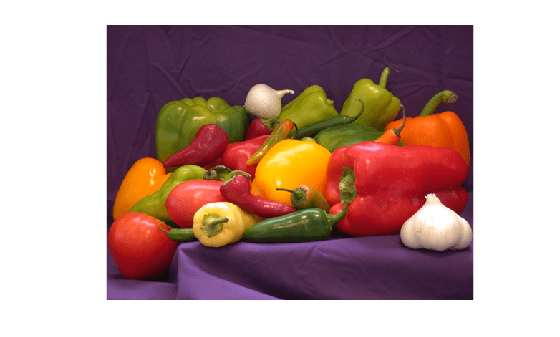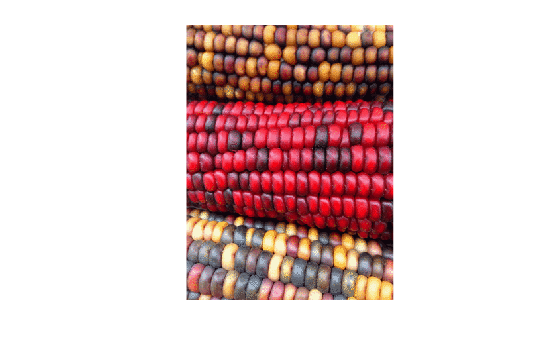# rgb2gray

## 语法

``I = rgb2gray(RGB)``
``newmap = rgb2gray(map)``

## 说明

``I = rgb2gray(RGB)` 将真彩色图像 `RGB` 转换为灰度图像 `I`。`rgb2gray` 函数通过消除色调和饱和度信息，同时保留亮度，来将 RGB 图像转换为灰度图。如果已安装 Parallel Computing Toolbox™，则 `rgb2gray` 可以在 GPU 上执行此转换。`

``newmap = rgb2gray(map)` 返回等同于 `map` 的灰度颜色图。`

## 示例

```RGB = imread('peppers.png'); imshow(RGB)``````I = rgb2gray(RGB); figure imshow(I)````[X,map] = imread('corn.tif');`

`imshow(X,map)````newmap = rgb2gray(map); imshow(X,newmap)```## 提示

• `rgb2gray` 支持使用 MATLAB® Coder™ 生成 C 代码。

## 算法

`rgb2gray` 通过计算 R、G 和 B 分量的加权和，将 RGB 值转换为灰度值：

```0.2989 * R + 0.5870 * G + 0.1140 * B ```

Rec.ITU-R BT.601-7 使用以下公式计算 E'y：

```0.299 * R + 0.587 * G + 0.114 * B ```

## 另请参阅

(Image Processing Toolbox) | (Image Processing Toolbox) | (Image Processing Toolbox) | | (Image Processing Toolbox)